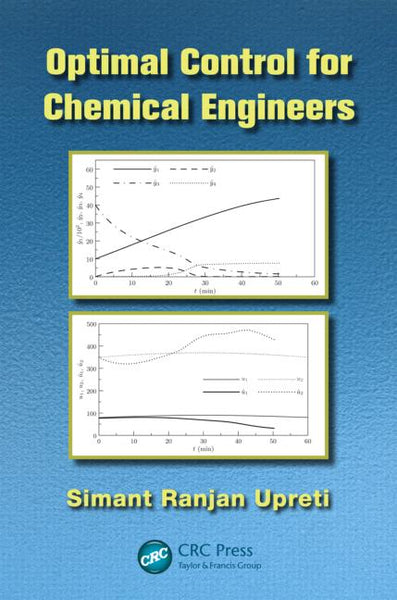# Optimal Control for Chemical Engineers

: In stock

: brown-tech

: 9781439838945

: Book

\$160.16

### Features

• Presents the necessary mathematical background using numerous examples
• Includes key mathematical results along with their derivations
• Focuses on problem formulation and solving
• Incorporates detailed computational algorithms and solutions of several example problems
• Devotes a whole chapter to optimal periodic control
• Contains end-of-chapter bibliographies and exercises

Solutions manual available with qualifying course adoption

### Summary

Optimal Control for Chemical Engineers gives a detailed treatment of optimal control theory that enables readers to formulate and solve optimal control problems. With a strong emphasis on problem solving, the book provides all the necessary mathematical analyses and derivations of important results, including multiplier theorems and Pontryagin’s principle.

The text begins by introducing various examples of optimal control, such as batch distillation and chemotherapy, and the basic concepts of optimal control, including functionals and differentials. It then analyzes the notion of optimality, describes the ubiquitous Lagrange multipliers, and presents the celebrated Pontryagin principle of optimal control. Building on this foundation, the author examines different types of optimal control problems as well as the required conditions for optimality. He also describes important numerical methods and computational algorithms for solving a wide range of optimal control problems, including periodic processes.

Through its lucid development of optimal control theory and computational algorithms, this self-contained book shows readers how to solve a variety of optimal control problems.

• Isbn-13 Digit:: 9781439838945